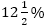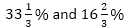IBPS RRB Quantitative Aptitude Quiz

Here, we are providing IBPS RRB Study Plan as there is left not enough time to deal in details. The questions asked in the quantitative aptitude section are calculative and very time-consuming. But once dealt with proper strategy, speed, and accuracy, this section can get you the maximum marks in the examination. Following is the Quantitative Aptitude Quiz to help you practice with the best of latest pattern questions.

Q1. Ravi bought two handbags each of Rs. 1200. He sold one bag at a profit ofand other at a loss of 25%. Find his overall profit percentage.

Q2. The cost of 16 valets is equal to selling price of 9 bags. If cost of four valets is equal to Rs. 60 and cost of two bag is Rs. 50 then what is the profit earned on a bag?

Q3. The marked price of a ceiling fan ismore than the marked price of a table fan. A shopkeeper sells the ceiling fan in Rs. 2400 by giving a discount of 20%. If table fan is sold at a discount of 20% and cost price of it is Rs. 1600, then, what is profit percentage earned on table fan?
12.5%
8.5%
12%
14%
10.5%

Q4. The ratio between cost price of one kg potato & one kg onion is 2 : 3. Retailer sells 4.5 kg potato in Rs. 72 and makes a profit ofcalculated at its selling price. If he sells one kg onion at the rate of Rs. 24, then find profit percentage earned on onion.

Q5. A wrist- watch is markedabove its cost price. The shopkeeper allows two successive discounts ofand and sells the watch in Rs. 600. Find the profit/loss (in rupees) earned on wrist- watch.
Rs. 48 profit
Rs. 48 loss
Rs. 36 loss
Rs. 36 profit
Rs. 96 loss

Q6. A person buys certain quantity of an article at Rs. 800. If he sells one –fourth at 20% loss, at what percent gain he should sell the remaining stock so as to make an overall 10% profit on total transaction?
12%
15%
18%
20%
None of these

Q7. The cost of pure milk is Rs. 16 per litre. On adding water, the mixture is sold at Rs. 15 per litre. In this way, the milkman earns 20% profit. What is the ratio of milk to water in the mixture?
25 : 1
7 : 25
15 : 1
1 : 15
25: 7

Q8. A shopkeeper has goods of worth Rs. 6000. He sold half of the goods at a gain of 12%. At what profit per cent should he sell the remaining half of the stock so that he gets 18% profit on the whole?
25%
24%
18%
8%
None of these

Q9. A trader marks his goods 30% above the cost price and gives a discount of 15% on the marked price. What gain% does he make?
10.5%
12%
10%
14.5%
16.5%

Q10. The labeled price of a product is Rs 750. If it is sold at a 20% discount and the dealer earns a 25% profit, what is the cost price?
Rs 550
Rs 450
Rs 435
Rs 480
Rs 520

Directions (11-15): What will come in place of the question mark (?) in the following questions?

Q11. (3325÷25) × (152÷16) =?
1269.4
1264.9
1265.3
1263.5
126.63

Q12.14
(196)²
–14
144
196

Q13.15
13
11/15
12
11

Q14. -15 -27 -88 -63 +266 -193 +255 =?
55
74
135
59
65

Q15. (2525×0.25÷5) ×7 =?
889.43
883.75
886.45
881.75
88.645

You May also like to Read: